# MIND召回介绍

## May 25, 2019

#

tmall在《Multi-Interest Network with Dynamic Routing for Recommendation at Tmall》开放了它们的召回算法。在matching stage上，提出了Multi-Interest Network with Dynamic routing (MIND)来处理用户的多样化兴趣。特别的，还基于capsule routing机制设计了一个multi-interest extractor layer，用于聚类历史行为和抽取多样化兴趣。另外，我们还开发了一种称为”label-aware attention”的技术来帮助学习具有多个向量的用户表示 。目前的效果要好于state-of-the-art的其它推荐方法。并在天猫APP的移动端主页上部署，会处理主要的在线流量。

# 1.介绍

tmall APP的主页如图1(左）所示，它占据着tmall APP的半数流量，并会部署RS来展示个性化的商品来满足客户个性化需求。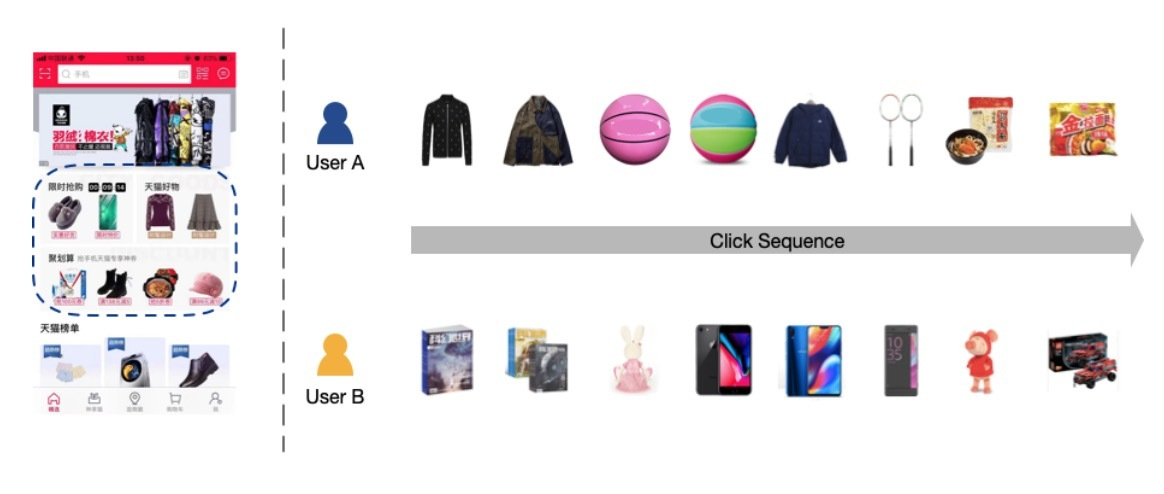• 1.为了捕获用户行为的多样化兴趣，我们设计了multi-interest extractor layer，它可以利用dyniamic routing来自适应地将用户历史行为聚合成用户表示向量。
• 2.通过使用由multi-interest extractor layer和一个新提出的label-aware attention layer生成的用户表示向量(vectors)，我们构建一个DNN来做个性化推荐。对比已存在的方法，MIND在多个公开数据集上和天猫上的效果要好一些。
• 3.为了在tmall上部署MIND，我们构建了一个系统来实现整个pipline，包括：data collecting、model training和online serving。部署的系统可以极大提升Tmall APP的ctr。

# 2.相关工作

User Representation。在推荐系统中，将users表示为vectors很常见。传统的方法会将用户偏好组合成由感兴趣的items[4,12,22]、keywords[5,8]和topics的vectors。随着分布式表示学习的出现，user embeddings可以通过NN获得。使用RNN-GRU来从时序阅读文档中学习user embeddings。从word embedding vectors中学习user embedding vectors，并将它们应用于推荐学术微博上。提出了一种新的CNN-based模型来显式学习和利用user embeddings。

Capsule Network。“胶囊（Capsule）”的概念，对一小部分neurons组合输出一整个vector，首次由2011年Hinton提出。用于替代BP，dynamic routing被用于学习capsules间连接的权重，通过利用EM算法来克服多种缺陷并达到较好的accuracy。它与CNN有两个主要不同之处，使得capsule networks可以编码在part和whole间的关系，这在CV和NLP中是很先进的。SegCaps证明，capsules可以成功建模目标的局部关系（spatial），比传统CNNs要好。研究了文本分类的capsule网络，并提出了3种策略来增强效果。

# 3.方法

## 3.1 问题公式化

• $I_u$表示与用户u交互的items集合（也称为：用户行为(user behavior)）
• $P_u$是用户u的基础profiles（比如：gender和age）
• $F_i$是target item的特征（比如：item id和category id）

MIND的核心任务是，学习一个函数，来将原始特征（raw features）映射到用户表示上，它可以公式化为：

$V_u = f_{user}(I_u, P_u)$

…(1)

$\vec{e}_i = f_{item}(F_i)$

…(2)

$f_{score}(V_u, \vec{e}_i) = \underset{1 \leq k \leq K}{max} \vec{e}_i^T \vec{e}_u^k$

…(3)

## 3.2 Embedding & Pooling Layer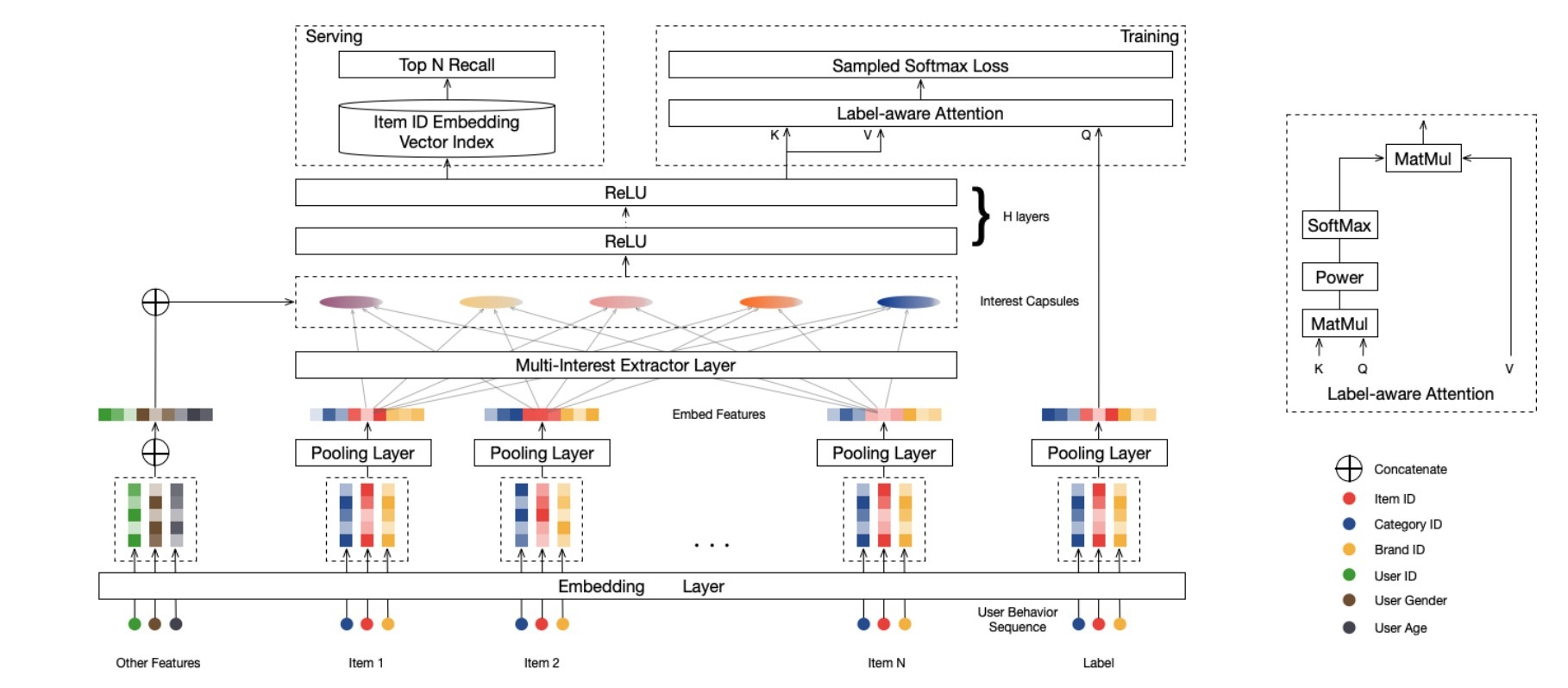## 3.3 Multi-Interest Extractor Layer

### 3.3.1 Dynamic Routing

$\vec{c}_i^l \in R^{N_l \times 1}, i \in \lbrace 1,\cdots,m \rbrace$

high-level capsules $j \in \lbrace 1, \cdots, n \rbrace$，它相应的向量为：

$\vec{c}_j^h \in R^{N_h \times 1}, j \in \lbrace 1, ..., n \rbrace$

$b_{ij} = (\vec{c}_j^h)^T S_{ij} \vec{c}_i^l$

…(4)

$\vec{z}_j^h = \sum\limits_{i=1}^m w_{ij} S_{ij} \vec{c}_i^l$

…(5)

$w_{ij} = \frac{exp (b_{ij})}{ \sum\limits_{k=1}^m exp (b_{ik})}$

…(6)

$\vec{c}_j^h = squash(\vec{z}_j^h) = \frac{\| \vec{z}_j^h \|^2}{ 1 + \| \vec{z}_j^h \|^2} \frac{\vec{z}_j^h}{ \| \vec{z}_j^h \|}$

…(7)

$b_{ij}$的值被初始化为0, routing process通常会重复三次以便收敛。当routing完成时，high-level capsule的值$\vec{c}_j^h$是确定的，可以被当作是next layers的inputs。

### 3.3.2 B2I Dynamic Routing

1.Shared bilinear mapping matrix.

• 一方面，用户行为是变长的，对tmall用户来说，范围从几十到几百不等，因而，使用固定的bilinear mapping matrix是可泛化推广（generalizable）的
• 另一方面，我们希望interest capsules位于相同的向量空间中，但不同的bilinear mapping matrice会将interest capsules映射到不同的向量空间上。从而，routing logit可以通过以下公式计算：
$b_{ij} = \vec{u}_j^T S \vec{e}_i, i \in I_u, j \in \lbrace 1, \cdots, \rbrace$

…(8)

• $\vec{e}_i \in R^d$表示behavior item i的embedding
• $\vec{u}_j \in R^d$表示interest capsule j的向量。
• bilinear mapping matrix $S \in R^{d \times d}$是跨每个(behavior capsules, interest capsules) pairs间共享的。

2.随机初始化routing logits

3.动态兴趣数(Dynamic interest number)

$K_u' = max(1, min(K, log_2(|I_u|)))$

…(9)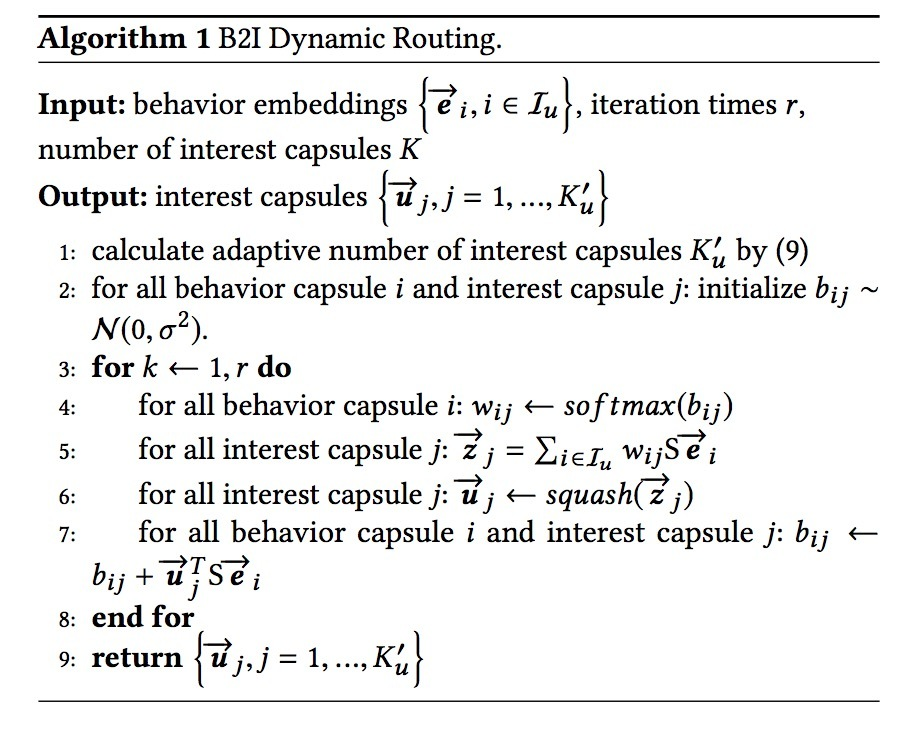## 3.4 Label-aware Attention Layer

\begin{align} \vec{v}_u & = Attention (\vec{e}_i, V_u, V_u) \\ & = V_u softmax(pow(V_u^T \vec{e}_i, p)) \end{align}

• pow表示element-wise指数操作符(exponentiation oprator)，pow(x,y)表示x的y次幂；
• p是一个可调参数，用于调节attention分布。当p接近0时，每个interest capsule趋向于接收偶数(even)attention。当p大于1时，随着p的增加，该值会大于点乘，会接受越来越多的权重。考虑到极限的情况，当p无穷大时，attention机制会变成一种hard attention，它会选中具有最大attention的值，并忽略其它。在我们的实验中，我们发现：使用hard attention会导致更快的收敛。

• $\vec{e}_i$表示target item i的embedding
• $V_u$：用户u的表示向量，共由K个interest capsules组成
• $\vec{v}_u$：user u对于item i的output vector

## 3.5 Training & Serving

$Pr(i | u) = Pr(\vec{e}_i | \vec{v}_u) = \frac{exp(\vec{v}_u^T \vec{e}_i)}{ \sum_{j \in I} exp(\vec{v}_u^T \vec{e}_j)}$

…(10)

$L = \sum\limits_{(u,i) \in D} log Pr(i|u)$

…(11)

## 3.6 与已存在方法的联系

DIN. DIN可以捕获用户的多样化兴趣，MIND和DIN具有相似的目标。然而，这两种方法在达成该目标以及应用上各不相同。为了处理多样化兴趣，DIN会在item级别使用一个attention机制，而MIND使用dynamic routing来生成interest capsules，并在interest level考虑多样性。再者，DIN关注在ranking stage处理上千的items，而MIND会解耦inferring用户表示和衡量user-item兼容性，使它应用于在matching stage上海量items的召回。

# 4.实验

## 4.2 超参数分析

routing logits的初始化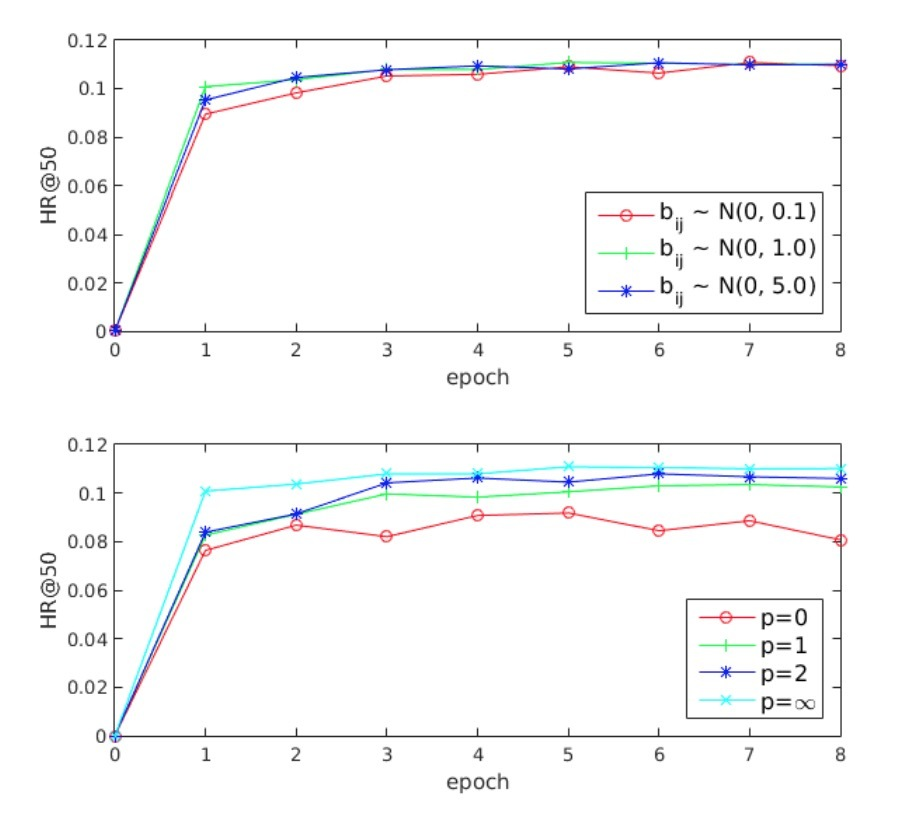## 4.3 在线实验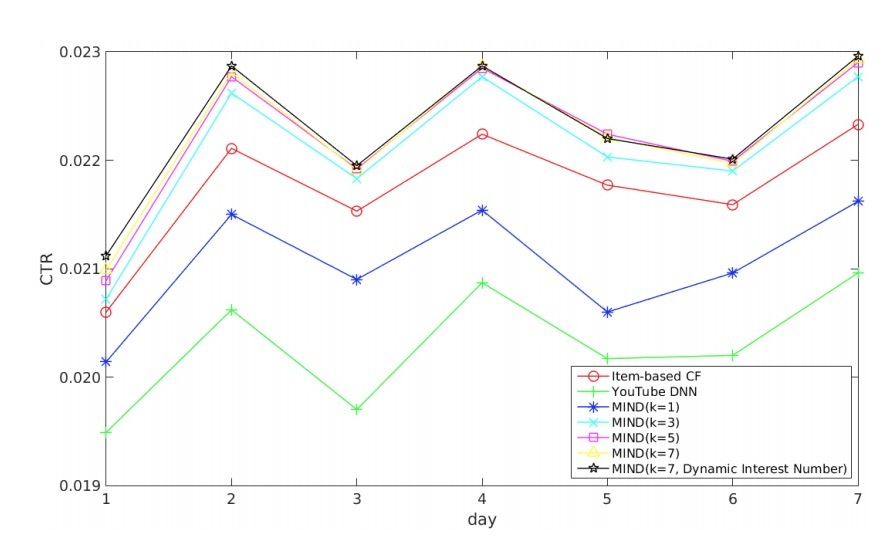• 2) 另一个显著的趋势是，MIND的效果会随着抽取的兴趣数的增加而变好（从1到5）。
• 3) 当抽取的兴趣数为5时，MIND的效果达到峰值，这之后，CTR保持数据，兴趣数达到7的提升可以忽略。
• 4) 使用动态兴趣数(dynamic interest number)的MIND与具有7个兴趣的MIND效果相当。

• 首先，对于Tmall，用户兴趣的最优数目是5-7, 这可以表示用户兴趣的平均多样性（diversity）。
• 第二，动态兴趣数机制并不能带来CTR增益，但在实验期间，我们意识到该scheme可以减少serving的开销，这有利于tmall这样的大规模服务，在实际上更易接受。

## 4.4 案例研究

### 4.4.1 耦合系数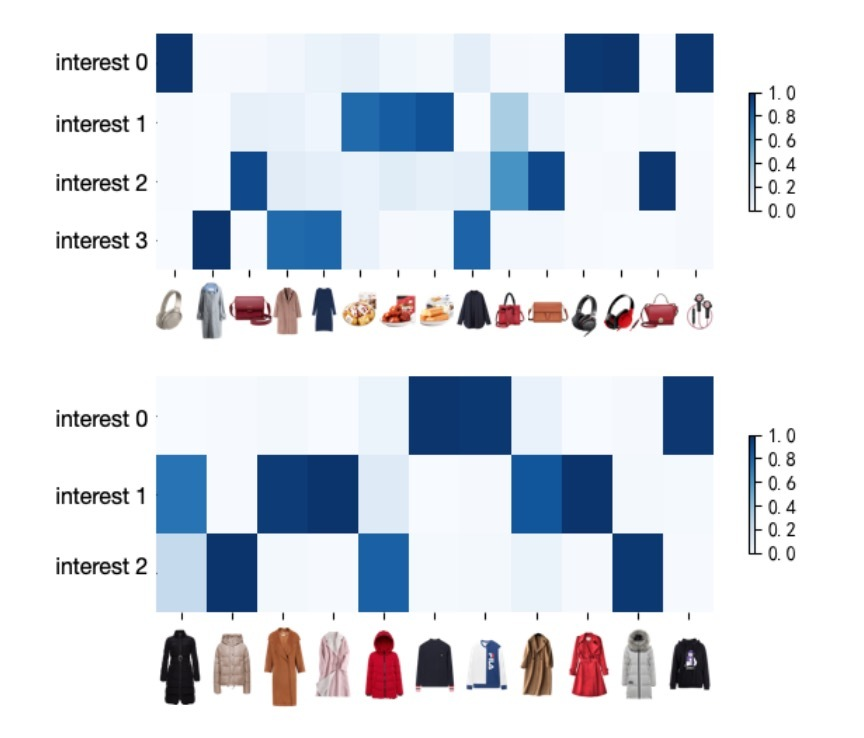### 4.4.2 item分布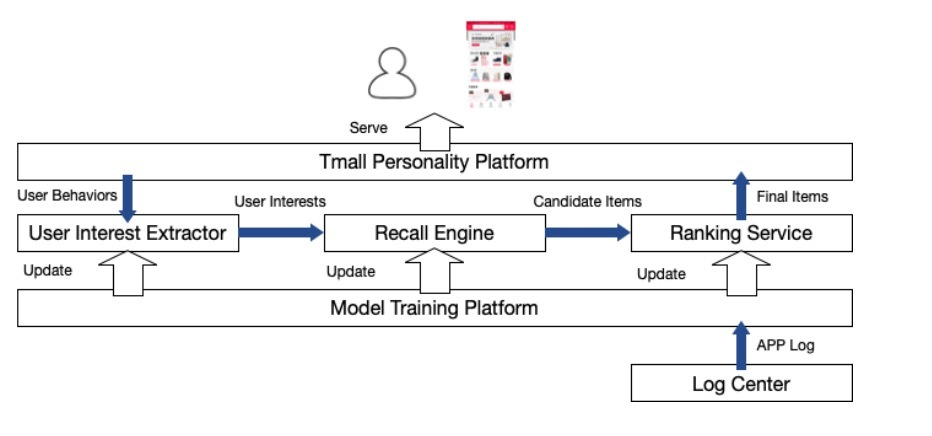# 5.系统部署# 参考

Updated on

### PLE介绍

tx在《Progressive Layered Extraction (PLE): A Novel Multi-TaskLearning (MTL) Model for Personalized Recommendations》提出了PLE模型：# 1.介绍个性化推荐在在线...… Continue reading

#### DTS介绍

Published on January 02, 2021

#### taobao Privileged Features Distillation介绍

Published on December 16, 2020